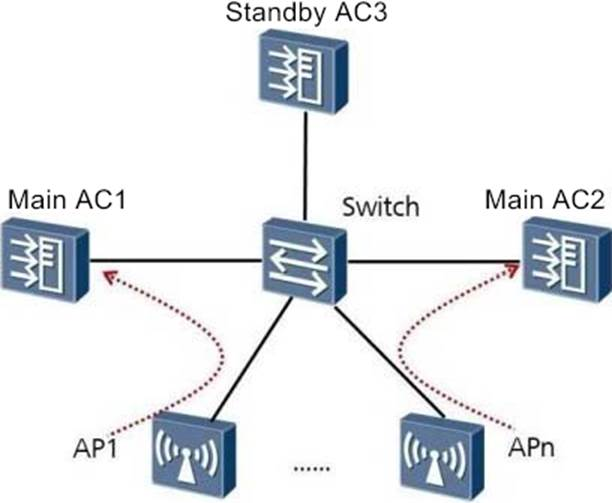# In the N+1 backup network as shown in the figure, AC3 is the backup AC of AC1 and AC2, the number of APs that are online on the main AC1 is 1024, the number of configured APs is 2048, and the number of APs that are online on the main AC2 is 512. Normally, what is the minimum number of APs allowed to access on AC3, and what is the minimum number of APs allowed to be configured on AC3?

In the N+1 backup network as shown in the figure, AC3 is the backup AC of AC1 and AC2, the number of APs that are online on the main AC1 is 1024, the number of configured APs is 2048, and the number of APs that are online on the main AC2 is 512. Normally, what is the minimum number of APs allowed to access on AC3, and what is the minimum number of APs allowed to be configured on AC3?A . 2048, 1024
B. 1024, 2048
C. 3072, 512
D. 1024, 2560